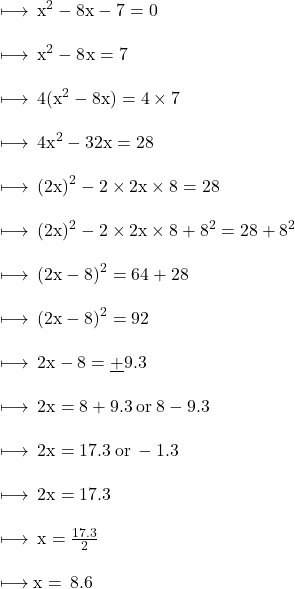## When solving the equation x2 – 8x – 7 = 0 by completing the square, which equation is a step in the process?

Question

When solving the equation x2 – 8x – 7 = 0 by completing the square, which equation
is a step in the process?

in progress 0
3 weeks 2021-08-23T23:21:23+00:00 1 Answers 1 views 0